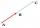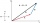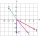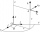# Vectors

For vector w is true: w = 2u-5v. Determine coordinates of vector w if u=(3, -1), v=(12, -10)

Correct result:

wx =  -54
wy =  48

#### Solution:

$w=2u-5v=\left(2\cdot 3+\left(-5\right)\cdot 12,2\cdot \left(-1\right)+\left(-5\right)\cdot \left(-10\right)\right)=\left(-54,48\right)$We would be pleased if you find an error in the word problem, spelling mistakes, or inaccuracies and send it to us. Thank you!Tips to related online calculators
Two vectors given by its magnitudes and by included angle can be added by our vector sum calculator.

## Next similar math problems:

• Vector - basic operationsThere are given points A [-9; -2] B [2; 16] C [16; -2] and D [12; 18] a. Determine the coordinates of the vectors u=AB v=CD s=DB b. Calculate the sum of the vectors u + v c. Calculate difference of vectors u-v d. Determine the coordinates of the vector w
• Scalar dot productCalculate u.v if |u| = 5, |v| = 2 and when angle between the vectors u, v is: a) 60° b) 45° c) 120°
• Linear independenceDetermine if vectors u=(-4; -5) and v=(20; 25) are linear Linear dependent.
• VectorDetermine coordinates of the vector u=CD if C[19;-7], D[-16,-5].
• Coordinates of vectorDetermine the coordinate of a vector u=CD if C(19;-7) and D(-16;-5)
• VectorsVector a has coordinates (8; 10) and vector b has coordinates (0; 17). If the vector c = b - a, what is the magnitude of the vector c?
• Vector sumThe magnitude of the vector u is 12 and the magnitude of the vector v is 8. Angle between vectors is 61°. What is the magnitude of the vector u + v?
• Coordinates of square verticesThe ABCD square has the center S [−3, −2] and the vertex A [1, −3]. Find the coordinates of the other vertices of the square.
• Scalar productCalculate the scalar product of two vectors: (2.5) (-1, -4)
• Vector equationLet’s v = (1, 2, 1), u = (0, -1, 3) and w = (1, 0, 7) . Solve the vector equation c1 v + c2 u + c3 w = 0 for variables c1 c2, c3 and decide weather v, u and w are linear dependent or independent
• Parallel and orthogonalI need math help in this problem: a=(-5, 5 3) b=(-2,-4,-5) (they are vectors) Decompose the vector b into b=v+w where v is parallel to a and w is orthogonal to a, find v and w
• Angle between vectorsFind the angle between the given vectors to the nearest tenth of a degree. u = (-22, 11) and v = (16, 20)
• Intersect and conjuctionLet U={1,2,3,4,5,6} A={1,3,5} B={2,4,6} C={3,6} Find the following. 1. )AUB 2. )A'UB'
• Unit vector 2DDetermine coordinates of unit vector to vector AB if A[-6; 8], B[-18; 10].
• Three vectorsThe three forces whose amplitudes are in ratio 9:10:17 act in the plane at one point so that they are in balance. Determine the angles of the each two forces.
• 3d vector componentThe vector u = (3.9, u3) and the length of the vector u is 12. What is is u3?
• LineLine p passing through A[-10, 6] and has direction vector v=(3, 2). Is point B[7, 30] on the line p?